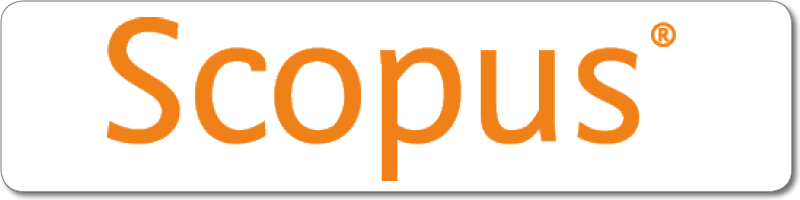| |
River Publishers Series in Mathematical, Statistical and Computational Modelling for Engineering

## Elementary Vector Calculus and Its Applications with MATLAB Programming Forthcoming

Authors:
Nita H. Shah, Head and Professor, Department of Mathematics, Gujarat University, India
Jitendra Panchal, Assistant Professor, Department of Applied Sciences & Humanities, Parul Institute of Engineering & Technology, Parul University, India

ISBN: 9788770223874
e-ISBN: 9788770223867

Price: \$ 132.00Distributed exclusively by Routledge (Taylor & Francis Group)Description:

Sir Isaac Newton, one of the greatest scientists and mathematicians of all time, introduced the notion of a vector to define the existence of gravitational forces, the motion of the planets around the sun, and the motion of the moon around the earth. Vector calculus is a fundamental scientific tool that allows us to investigate the origins and evolution of space and time, as well as the origins of gravity, electromagnetism, and nuclear forces. Vector calculus is an essential language of mathematical physics, and plays a vital role in differential geometry and studies related to partial differential equations widely used in physics, engineering, fluid flow, electromagnetic fields, and other disciplines. Vector calculus represents physical quantities in two or three-dimensional space, as well as the variations in these quantities. The machinery of differential geometry, of which vector calculus is a subset, is used to understand most of the analytic results in a more general form. Many topics in the physical sciences can be mathematically studied using vector calculus techniques. This book is designed under the assumption that the readers have no prior knowledge of vector calculus. It begins with an introduction to vectors and scalars, and also covers scalar and vector products, vector differentiation and integrals, Gauss's theorem, Stokes's theorem, and Green's theorem. The MATLAB programming is given in the last chapter.

This book includes many illustrations, solved examples, practice examples, and multiple-choice questions.

Keywords:

Vectors, scalars, vector differentiation, vector integration, MATLAB programming